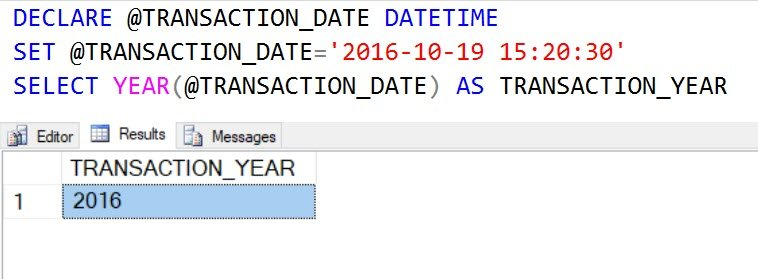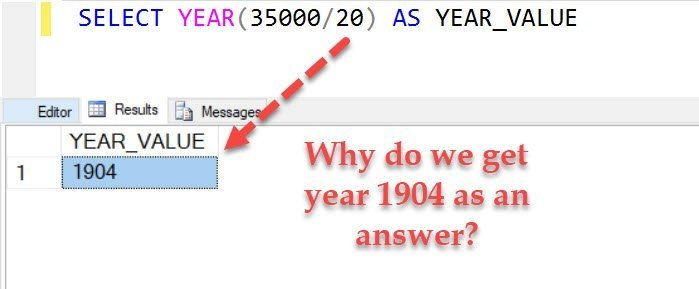# SQL SERVER – Puzzle – How Does YEAR Function Work?

There are many different datetime related functions such as DAY, MONTH, YEAR, DATEDIFF, etc available in SQL Server. For example, YEAR function can be used to extract year value from a date.

Let me show you a simple example

```DECLARE @TRANSACTION_DATE DATETIME
SET @TRANSACTION_DATE='2016-10-19 15:20:30'
SELECT YEAR(@TRANSACTION_DATE) AS TRANSACTION_YEAR```

When you run above script, it gives us following resultset.### Puzzle – Year Function

Now let us see an exciting mystery which involves Year Function.

Now execute the following SELECT statement

`SELECT YEAR(35000/20) AS YEAR_VALUE`When you ran the above script, it gives us a result as the year 1904.

This is indeed a strange result. As you can see that 35000/20 is not a valid date value. The matter of the fact 35000/20 is results in value 1750.

Puzzle: How does SQL Server consider this as a valid date and return year value as 1904?

Please leave your answers in the comment sections. I will be publishing all the valid answers next week same time. I have so far posted quite a many puzzles, I believe this one is the most challenging puzzle.

I suggest you share this with your friends who know SQL and see if they can solve this or not. I promise you that the answer to this puzzle is straightforward, once you know the trick behind it.

Reference: Pinal Dave (https://blog.sqlauthority.com)

#### Related Posts

•Vipin Jain
March 13, 2018 9:17 am

SQL Server supports the 1900 date system, in this when you enter a date, the date is converted into a serial number that represents the number of elapsed days since January 1, 1900. So if we are doing Select year(35000/20) = 1904. However, the serial number is year(1750), So now 1750 days (4 years) will be added in 1900 year and answer will be 1904.
I hope, my answer is correct.

•iambdot
March 13, 2018 11:43 pm

I agree with this answer. 1750 days from 01/01/1900 is 10/17/1904

•Jeet
March 15, 2018 3:49 pm

Wow.. got to know something interesting :)

•aungko
March 13, 2018 10:04 am

-For this puzzle, I understand that YEAR() function access argument type is (a time, date, smalldatetime, datetime, datetime2, or datetimeoffset) value and return type value is integer and default value is base year 1900.
If the argument value is integer in YEAR() function where integer argument value is divided by 365 days to get only integer value and sum with base year so final result is 1904.

select YEAR(35000/20)
select YEAR(1750)

(Integer Argument Value Divided by 365) + Base Year = Result
(1750/365) = 4 1900 1904

– You can see this script to view result value.
SELECT YEAR(2554) AS ‘Year’ –Does not include in limitted argument type (a time, date, smalldatetime, datetime, datetime2, or datetimeoffset)
SELECT YEAR(‘2554-10-01’) AS ‘Year’ — Within limitted argument type

select YEAR(2554) AS ‘Six Year’
select YEAR(2189) AS ‘Five Year’
select YEAR(1824) AS ‘Four Year’
select YEAR(1459) AS ‘Three Year’
select YEAR(1094) AS ‘Two Year’
select YEAR(729) AS ‘One Year’

•March 13, 2018 11:46 am

More interesting is that, when we run this in SQL Server 2014 then it returns 1900

•sabin
March 13, 2018 11:53 am

Because is adding the number 1750 days to the year 0 (1900 – 01 – 01 )

select 35000/20 –1750

•sabin
March 13, 2018 11:56 am

select cast(35000/20 as datetime) , year (cast(35000/20 as datetime))

•Kees de Boer
March 13, 2018 12:58 pm

Datetime counts the number of days since 1900 with the time as a fraction of the number.
32000/20 yields 1750, which is 4 * 364 + 294 (1900 is not a leapyear) . 1904 is a lea year so day number 294 is Oct 15, 1904. As the called function only gives the year as an integer, 1904 is the logical answer.
There very few programming languages that store a date as a real datetype. Most store dates as thenumber of days since some beginnen point.
P.e. in SPSS a date is the number of seconds since Oct. 14, 1582…

•March 13, 2018 2:52 pm

May be Unknown date

•Sanjay Monpara
March 13, 2018 7:11 pm

in sql server, 0 means date 01-01-1900
so 35000/20 = 1750 means 1750th day from 01-01-1900
that’s 1904-10-17
so YEAR(35000/20) = 1904

•Ayan
March 13, 2018 8:25 pm

YEAR(35000/20) OR YEAR(1750) will return 1904.

Reason –

YEAR() without a date value returns 1900.
Now, when we are giving YEAR(1750) SQL Server is
“actually” counting the number of years. The number of years is 1750 / 365 (~ 4 years and some month) which rounds up to 4. So basically , SQL Server is counting the number of years from ‘0’ days to ‘1750’ days. Hence, the output is showing up as 1904.

•thesqlist
March 13, 2018 8:26 pm

The YEAR function converts the 35000/20 expression to a datetime value, which has the result 1904-10-17 00:00:00.000, hence the result of 1904 for the YEAR(35000/20):

SELECT cast(35000/20 as datetime)
———————–
1904-10-17 00:00:00.000

•March 14, 2018 3:05 pm

Year function has an argument of date datatype.
Internally SQL Server store a date datatype as an integer representing the days after the date 01-01-1900.

So the result of 35000/2= 1750 is the number of days after the 01-01-1900.
select dateadd (“dd”, 1750, ‘19000101’) . The result is 1904-10-17 00:00:00.000.

Year(‘1904-10-17 00:00:00.000’)= 1904

•James Curran
March 15, 2018 12:38 am

As only briefly mentioned in one post above. a DateTime value is actually a numeric (non-integer) number, with the whole number portion the number of days, and the fraction, the partial days (i.e, the time)
So that 1750.0 is midnight on 1904-10-17, and 1750.5 is noon on that date, and 1750.999305555555555555 is one minute before midnight at the end of the day.

•bumhwaklee
March 16, 2018 5:36 pm

Empty(”) parameter is 1900 in YEAR() function. And Empty(”) + 365 parameter is 1901 in YEAR() function.
So, Empty(”) + 1750 is 1904 in YEAR() function.

•MKumar
April 9, 2018 7:10 pm

In SELECT YEAR(35000/20) AS YEAR_VALUE query, the argument value will be evaluated to YEAR(1750). Now, sql server will try to resolve this integer value to a time, date, smalldatetime, datetime, datetime2, or datetimeoffset (as per docs.microsoft.com). It will do implicit type cast something like- CAST(1750 as datetime), which will result in value- 1904-10-17 00:00:00.000. Now YEAR function will take out Year part.

•Esther Xaviour
May 18, 2018 11:50 pm

35000/20=1750. Since the Year field is in the Date format, 1750 is equivalent to 10/15/1904. So the Year function is extracting the year 1904.

•hardikumarawalardik
June 14, 2019 5:06 pm

select year (35000/20) as year_value

when we run this query we found values 1904

Reason:
When we execute sql query
first we get value select 35000/20 = 1750 and its Int value when it convert into year then

select year(dateadd(day,1750,’19000101′)) then we found 1904 ( default date is 19000101 in SQL )